# Physical Chemistry : Freezing Point

## Example Questions

### Example Question #2 : Colligative Properties

When 20 grams of a solute is added to a liter of water, the freezing point becomes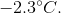What is the molar mass of the solute?

Assume that the solute does not make ions in solution.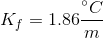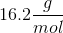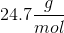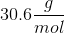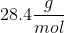Explanation:

We can use the freezing point depression equation in order to determine the molar mass of the unknown solute.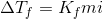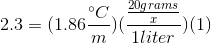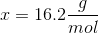### All Physical Chemistry Resources